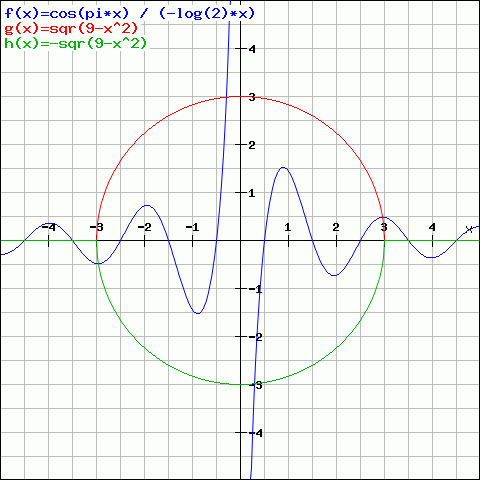Welcome Guest!

# Sample Plot Page

For page specific messages
For page author info

• create images of function plots using the plotting tool given below,
• save the plot images in your file-space, and
• insert the images from file-space into any content you edit.

### Exclude node summary :

nx^2

The above plot image has been created by plotting 3 functions -

1. f(x) = cos(pi*x)/(-log(2)*x)
2. g(x) = sqr(9-x^2)
3. h(x) = - sqr(9-x^2)

### Introduction

The function plotter is an embedded tool that appears  when you are creating or editing a Plot page. The plotter appears with its separate toolbar below the main content. You can plot 3 items simultaneously. These items could be functions, derivatives of functions or integrals of functions.

### Two modes

There are two main ways to use it, which are related to its two modes (see toolbar) -

1. create plots and save the images for inserting them later in your content, (Form Mode)
2. write  tutorials with step-by-step notes for learners to read, reproduce plots and try new things (Note Mode)

### Plot properties

While the Note Mode is suitable for instructions, the Form Mode gives more control. For example,

• Plot range and plot style for each of the 3 items can be set independently.
• Modify display properties, choose different types of X- and Y-axis scales. (Choose which quadrant to show.)
• Save plot in 3 image formats - GIF, JPG or PNG. (The images are saved in your file-space under "plots" folder.)